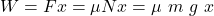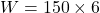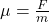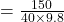## A horizontal force of 150 N is used to push a 40.0-kg packing crate a distance of 6.00 m on a rough horizontal surface. If the crate moves a

Question

A horizontal force of 150 N is used to push a 40.0-kg packing crate a distance of 6.00 m on a rough horizontal surface. If the crate moves at constant speed, find (a) the work done by the 150-N force and (b) the coefficient of kinetic friction between the crate and surface.

in progress 0
5 months 2021-08-26T12:43:55+00:00 1 Answers 52 views 0

## Answers ( )

a. 900 J

b. 0.383

Explanation:

According to the question, the given data is as follows

Horizontal force = 150 N

Packing crate = 40.0 kg

Distance = 6.00 m

Based on the above information

a. The work done by the 150-N force is= 900 J

b. Now the coefficient of kinetic friction between the crate and surface is= .383

We simply applied the above formulas so that each one part could calculate